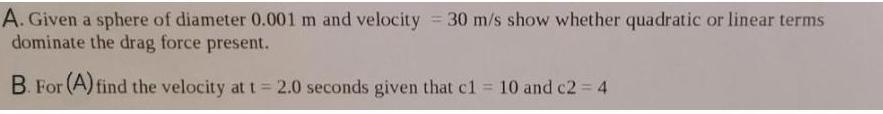Question:

# Given a sphere of diameter 0.001 m and velocity = 30 m/s

Last updated: 7/10/2022Given a sphere of diameter 0.001 m and velocity = 30 m/s show whether quadratic or linear terms dominate the drag force present. For (A) find the velocity at t = 2.0 seconds given that c1 = 10 and c2 = 4